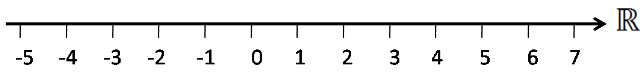The "Real Numbers" are all the "locations" on the number line.There are the rational numbers and the irrational numbers. Interestingly, between every pair of rational numbers there are infinitely many irrational numbers, and between every pair of irrational numbers there are infinitely many rational numbers.

The real numbers can be counter-intuitive.

The reals can be constructed via